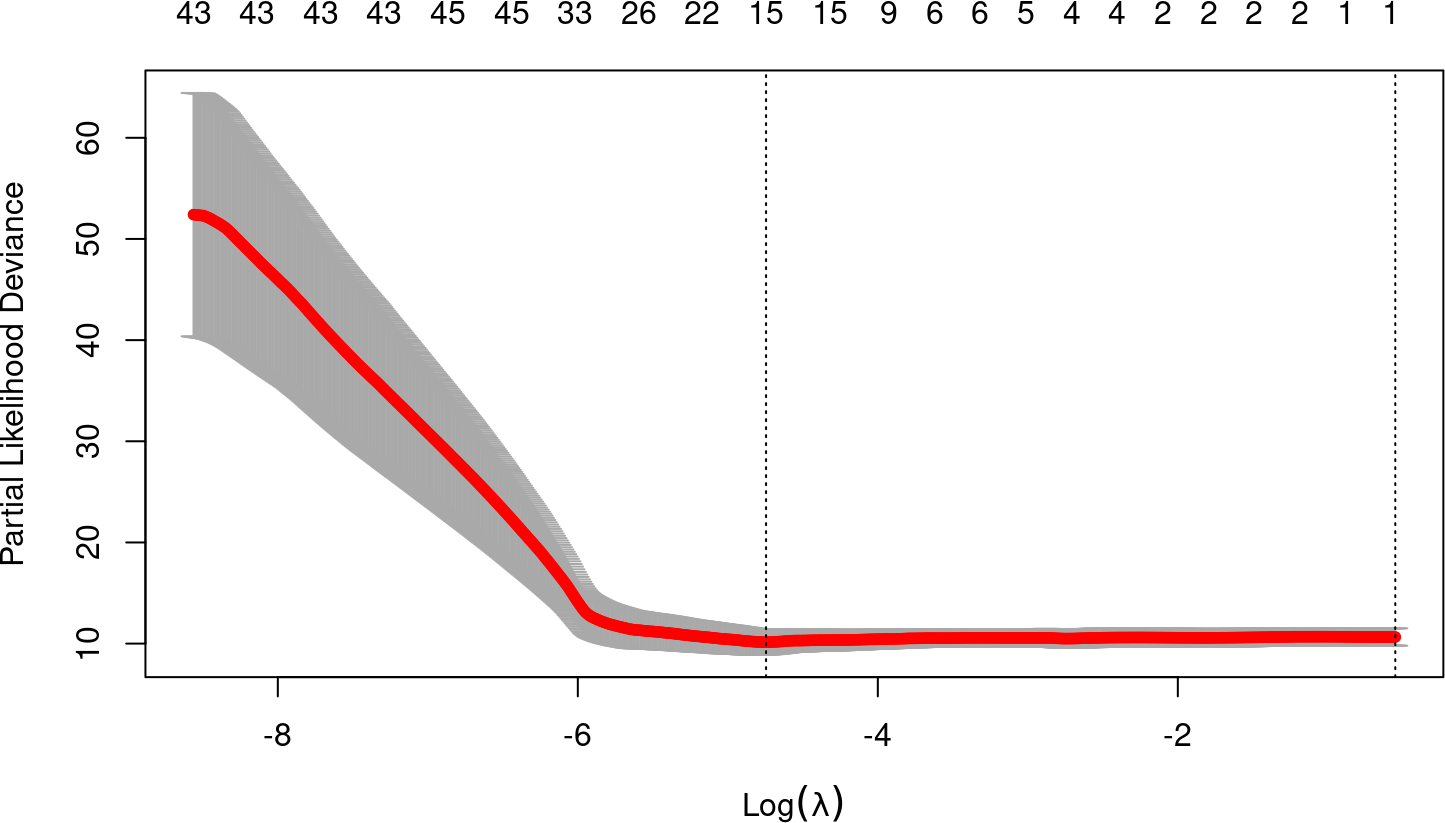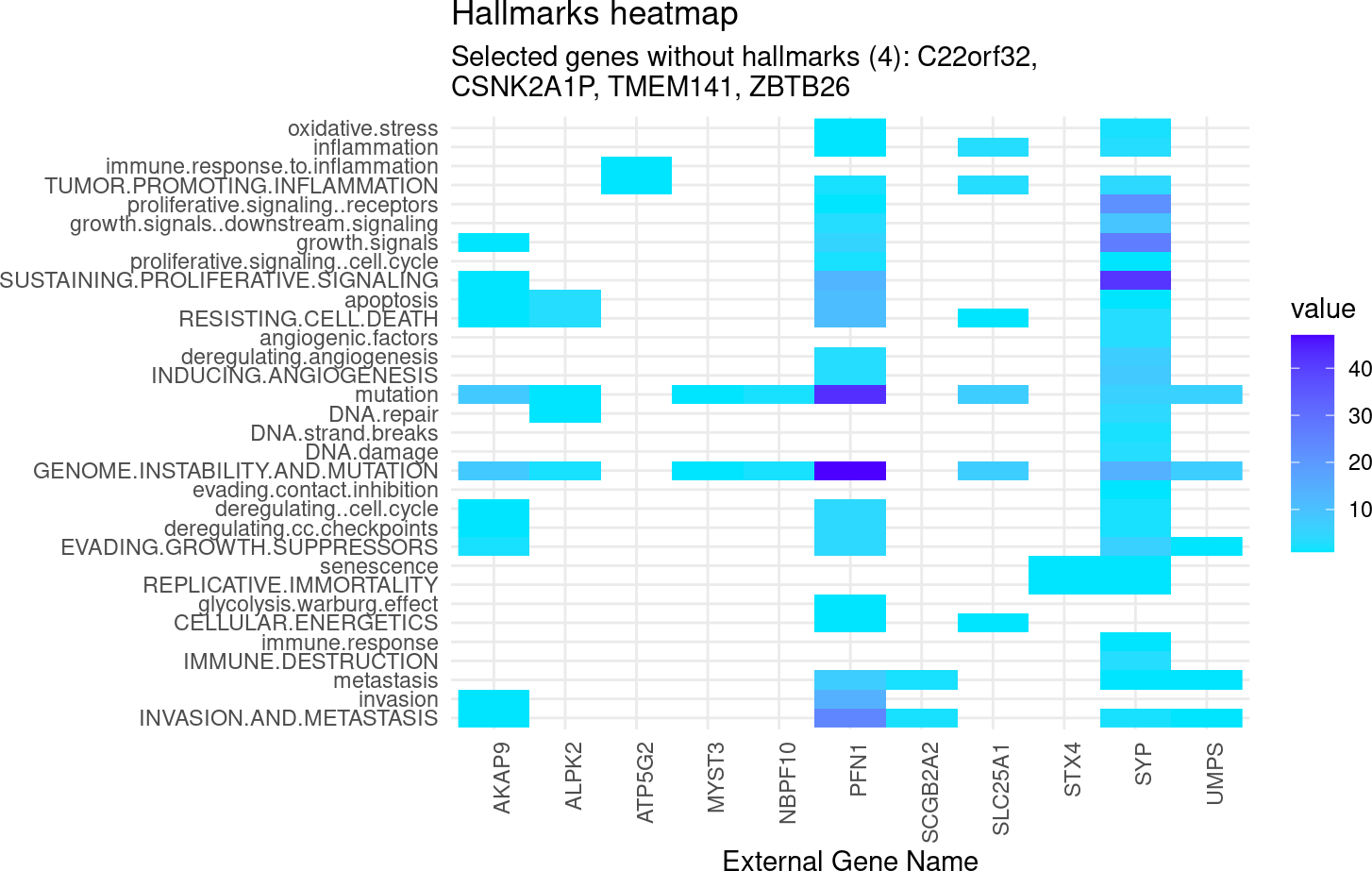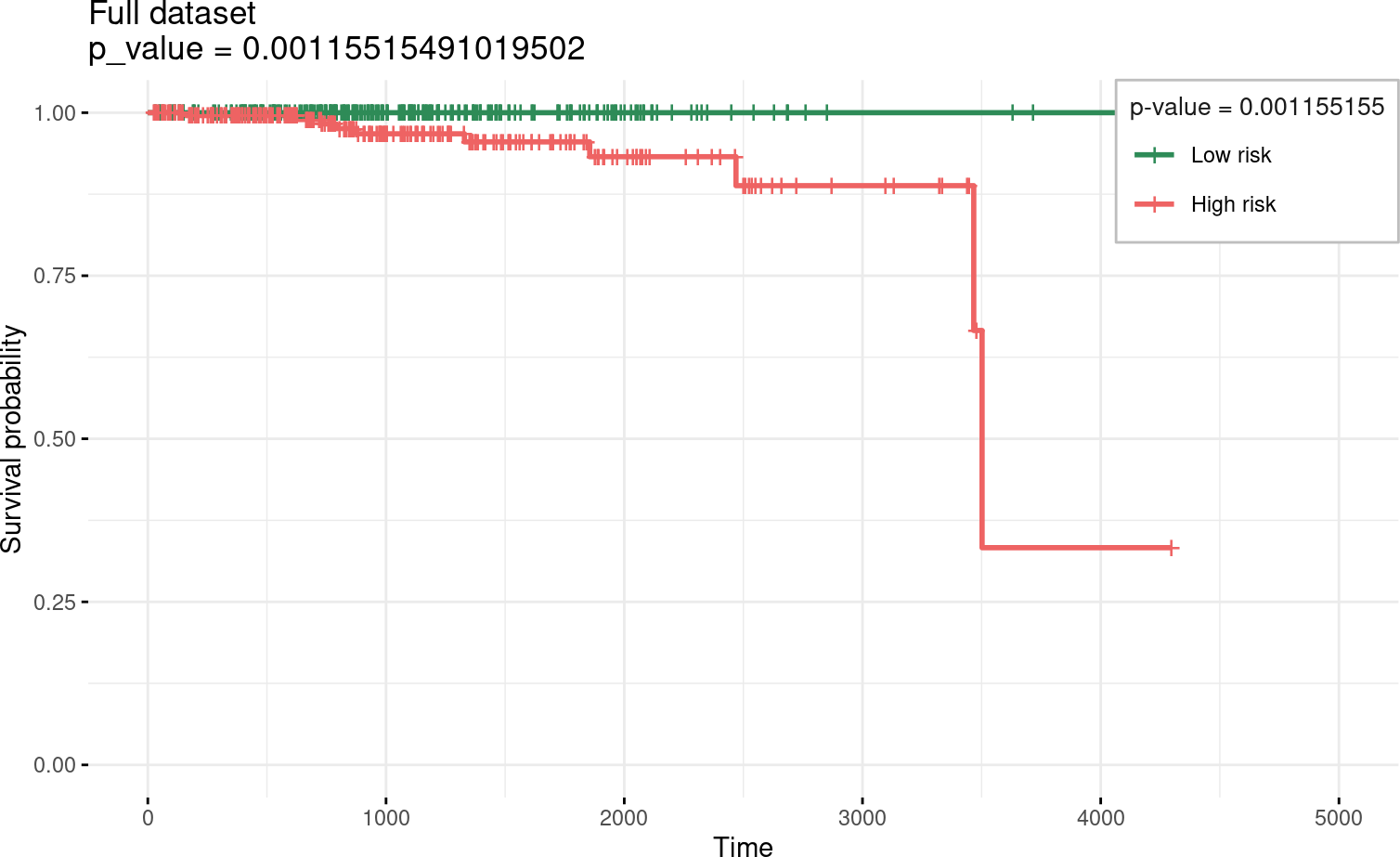# 1 Instalation

if (!require("BiocManager"))
install.packages("BiocManager")
BiocManager::install("glmSparseNet")

# 2 Required Packages

library(dplyr)
library(ggplot2)
library(survival)
library(loose.rock)
library(futile.logger)
library(TCGAutils)
#
library(glmSparseNet)
#
# Some general options for futile.logger the debugging package
.Last.value <- flog.layout(layout.format('[~l] ~m'))
.Last.value <- loose.rock::show.message(FALSE)
# Setting ggplot2 default theme as minimal
theme_set(ggplot2::theme_minimal())

The data is loaded from an online curated dataset downloaded from TCGA using curatedTCGAData bioconductor package and processed.

To accelerate the process we use a very reduced dataset down to around 100 variables only (genes), which is stored as a data object in this package. However, the procedure to obtain the data manually is described in the following chunk.

prad <- curatedTCGAData(diseaseCode = "PRAD", assays = "RNASeq2GeneNorm",
version = '1.1.38', dry.run = FALSE))

Build the survival data from the clinical columns.

• Selects only primary solid tumour samples
• Merge survival times for patients, which have different columns in case they are alive or dead.
• Build two matrix objects that fit the data xdata and ydata
# keep only solid tumour (code: 01)

# Get survival information
ydata.raw <- colData(prad.primary.solid.tumor) %>% as.data.frame %>%
# Find max time between all days (ignoring missings)
rowwise %>%
mutate(time = max(days_to_last_followup, days_to_death, na.rm = TRUE)) %>%
# Keep only survival variables and codes
select(patientID, status = vital_status, time) %>%
# Discard individuals with survival time less or equal to 0
filter(!is.na(time) & time > 0) %>% as.data.frame

# Set index as the patientID
rownames(ydata.raw) <- ydata.raw$patientID # keep only features that have standard deviation > 0 xdata.raw <- xdata.raw[TCGAbarcode(rownames(xdata.raw)) %in% rownames(ydata.raw),] xdata.raw <- xdata.raw %>% { (apply(., 2, sd) != 0) } %>% { xdata.raw[, .] } %>% scale # Order ydata the same as assay ydata.raw <- ydata.raw[TCGAbarcode(rownames(xdata.raw)), ] set.seed(params$seed)
small.subset <- c(geneNames(c('ENSG00000103091', 'ENSG00000064787',
'ENSG00000119915', 'ENSG00000120158',
'ENSG00000114491', 'ENSG00000204176',
'ENSG00000138399'))$external_gene_name, sample(colnames(xdata.raw), 100)) %>% unique %>% sort xdata <- xdata.raw[, small.subset[small.subset %in% colnames(xdata.raw)]] ydata <- ydata.raw %>% select(time, status) # 4 Fit models Fit model model penalizing by the hubs using the cross-validation function by cv.glmHub. set.seed(params$seed)
fitted <- cv.glmHub(xdata, Surv(ydata$time, ydata$status),
family  = 'cox',
nlambda = 1000,
network = 'correlation',
network.options = networkOptions(cutoff = .6,
min.degree = .2))

# 5 Results of Cross Validation

Shows the results of 100 different parameters used to find the optimal value in 10-fold cross-validation. The two vertical dotted lines represent the best model and a model with less variables selected (genes), but within a standard error distance from the best.

plot(fitted)## 5.1 Coefficients of selected model from Cross-Validation

Taking the best model described by lambda.min

coefs.v <- coef(fitted, s = 'lambda.min')[,1] %>% { .[. != 0]}
coefs.v %>% {
data.frame(ensembl.id  = names(.),
gene.name   = geneNames(names(.))$external_gene_name, coefficient = ., stringsAsFactors = FALSE) } %>% arrange(gene.name) %>% knitr::kable() ensembl.id gene.name coefficient AKAP9 AKAP9 AKAP9 0.2616307 ALPK2 ALPK2 ALPK2 -0.0714527 ATP5G2 ATP5G2 ATP5G2 -0.2575987 C22orf32 C22orf32 C22orf32 -0.2119992 CSNK2A1P CSNK2A1P CSNK2A1P -1.4875518 MYST3 MYST3 MYST3 -1.6177076 NBPF10 NBPF10 NBPF10 0.4507147 PFN1 PFN1 PFN1 0.4161846 SCGB2A2 SCGB2A2 SCGB2A2 0.0749064 SLC25A1 SLC25A1 SLC25A1 -0.8484827 STX4 STX4 STX4 -0.1690185 SYP SYP SYP 0.2425939 TMEM141 TMEM141 TMEM141 -0.8273147 UMPS UMPS UMPS 0.2214068 ZBTB26 ZBTB26 ZBTB26 0.3696515 ## 5.2 Hallmarks of Cancer geneNames(names(coefs.v)) %>% { hallmarks(.$external_gene_name)$heatmap }## 5.3 Survival curves and Log rank test separate2GroupsCox(as.vector(coefs.v), xdata[, names(coefs.v)], ydata, plot.title = 'Full dataset', legend.outside = FALSE) ##$pvalue
##  0.001155155
##
## $plot## ##$km
## Call: survfit(formula = survival::Surv(time, status) ~ group, data = prognostic.index.df)
##
##             n events median 0.95LCL 0.95UCL
## Low risk  249      0     NA      NA      NA
## High risk 248     10   3502    3467      NA